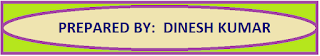### Common Errors in Secondary Mathematics

Common Errors Committed  by the  Students  in Secondary Mathematics   Errors  that students often make in doing secondary mathematics  during their practice and during the examinations  and their remedial measures are well explained here stp by step.  Some Common Errors in Mathematics

# Math Assignment  Class XII  Ch -07 | Integrals

Extra questions of chapter 07 Integrals class XII  with answers and  hints to the difficult questions, strictly according to the CBSE Board syllabus. Important and useful math. assignment for the students of class XII

## MATHEMATICS ASSIGNMENT OF EXTRA QUESTION

STRICTLY ACCORDING TO THE PREVIOUS CBSE BOARD SAMPLE QUESTION PAPERS
FROM 2018 TO 2022

Find the following integrals

Question 1

Question 2

Question 3

Question 4

Question 5

Solution Hint :  x2sinx is an odd function so this integral equal to zero

Question 6

Answer:  I = ex(1 - cot x) + C

Solution Hint

f(x) = 1 - cot x   ⇒  f ' (x) = cosec2

I = ex(1 - cot x)+C

Question 7

Question 8

Solution Hint

Now putting 1 - tan x = t

Question 9

Solution Hint

Question 10

Evaluate:

Solution Hint
Taking '-' common from the denominator and then applying method of completing the square.

Question 11

Evaluate:

Solution Hint:

......(1)

..... (2)

Adding equation (1) and equation (2) we get

Question 12

Evaluate:

Solution Hint

x - 1 = 0 when x = 1, so given integral becomes

Integrate and putting the limit we get  I = 5

Question 13

Question 14

Solution Hint

Now integrating the first integral by parts we get

Question 15

...... (1)

Solution Hint

Putting cos2x = t   ⇒  - 2 cosx sinx dx = dt  ⇒  Sin2x dx = - dt

Putting these values in equation (1) we get

Question 16

Solution Hint:  Factorise the given function we get

x(x - 1)(x - 2) = 0 ⇒ x = 0, 1, 2

Given integral can be written as

Find the integrals and putting the limits we get

Question 17

Solution Hint:

Putting x2 = t then use partial fraction  and then integrate

Question 18

Answer:  tan x - tan-1x + C

Solution Hint:

Adding and subtracting '1' in the numerator we get

Separating the denominator we get

I = tan x - tan-1x + C

Question 19

Solution Hint

Separating the denominator we get

Question 20

Solution Hint

Taking x4 common from the numerator we get

............. (1)

Differentiating both side we get

Putting all these values in eqn. (1) we get

Question 21

Solution Hint

I = I1 + I2

Since Iis an odd function so I1 = 0

Since is an even function so

⇒ I = 2log2
Question 22

Solution Hint:

Multiply and divide  by x we get

Now putting x2 = t   ⇒  2xdx = dt   ⇒ xdx = dt/2  we get

Using partial fraction to solve this integral

Solving these fractions we get,  A = 1, B = 0, C = - 2 and the given integral becomes

Question 23

Solution Hint

Question 24

Solution Hint

Putting sin x = t  ⇒ cosx dx = dt  we get

Now using partial fraction we get

Now putting  t = sinx we get

Question 25

Solution Hint

⇒ f(x) = -f(x) ⇒ f(x) is an odd function so

⇒ g(-x) = g(x) ⇒ g(x) is an even function so

Now given integral becomes

Divide  numerator and denominator by Cos2x we get

Putting  tan x = t   ⇒   sec2x dx = dt  we get

Integrating this and putting the limit we get

Question 26

Ans: 1
Solution Hint

Question 27

Ans:

Question 28

Ans:  sin-1(sin x - cos x)

Solution Hint

Putting  sin x - cos x = t    (sin x + cos x)dx = dt

Squaring sin x - cos x = t on both side we get:  sin2x = 1- t2

Making all substitution we get

Question 29

Question 30

Solution :

##THANKS FOR YOUR VISIT

1.Keep going . Very good work.